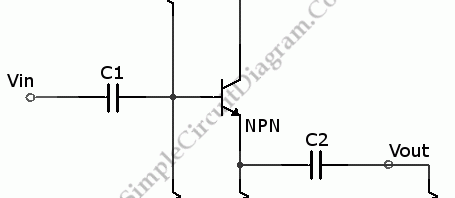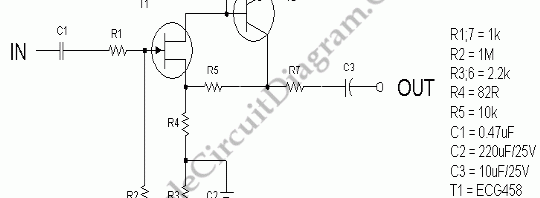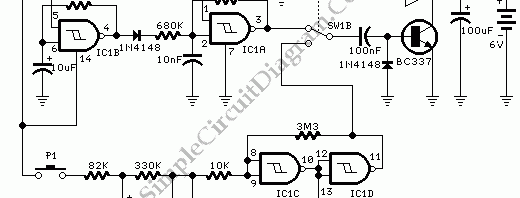## Transistor Emitter-Follower BufferFor a voltage source, an emitter follower can serve as a buffer. The voltage divider on the left is so strongly affected by the value of the load resistor make it a poor voltage source. The same voltage divider on the right added with a transistor buffer will supply power to keep the voltage constant over its range of operation. […]

## NPN Transistor Common Collector AmplifierThis is circuit of NPN Common Collector Amplifier. This circuit can be used for impedance matching. This circuit similar to the Emitter Follower circuit because the emitter of this circuit is follows the base minus a diode drop. This circuit has voltage gain of one because the output voltage is forced to follow about 0.6V below the signal input. Here […]

## JFET Low Noise PreampNoise, in amplifier circuit, is generated intrinsically by each components inside the circuit. Resistor, capacitor, and semiconductor generate noises. By simplifying the design of a circuit, we can minimize the noise especially if we can push the number of components to the lowest count. Here is one example of such design. The gain of this low noise amplifier is determined […]